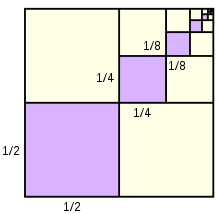# Consecutive 46781

We get three consecutive GP members if we subtract the same number from 33, 45, and 63. Determine this GP and calculate its fifth member.

a1 =  24
q =  1.5
a5 =  121.5

### Step-by-step explanation:Did you find an error or inaccuracy? Feel free to write us. Thank you!

Tips for related online calculators
Are you looking for help with calculating roots of a quadratic equation?
Do you have a linear equation or system of equations and looking for its solution? Or do you have a quadratic equation?